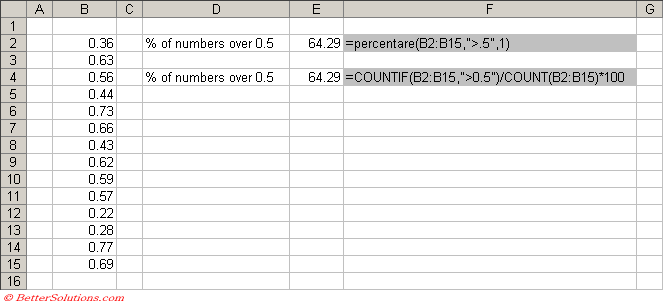### PERCENTAGEIF

Returns the percentage of numbers in a range that meet a particular condition.

`'rgeValues - The range of values.'sCondition - The condition you want to use.'iDecimalPlaces - The number of decimal places you want the percentage returned as.Public Function PERCENTAGEIF(ByVal rgeValues As Range, _                            ByVal sCondition As String, _                            ByVal iDecimalPlaces As Integer) As Double    PERCENTAGEIF = VBA.Round((Application.WorksheetFunction.CountIf(rgeValues, sCondition) / _                  Application.WorksheetFunction.Count(rgeValues)) * 100, iDecimalPlaces) End Function `